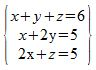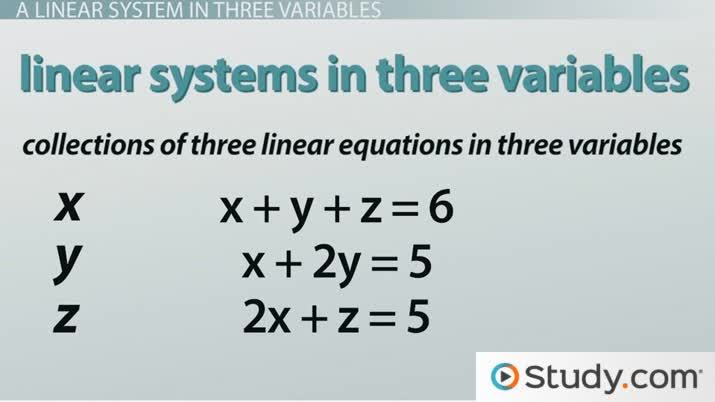How to Solve a Linear System in Three Variables With a Solution

Lesson Transcript
Instructor: Yuanxin (Amy) Yang Alcocer

Amy has a master's degree in secondary education and has been teaching math for over 9 years. Amy has worked with students at all levels from those with special needs to those that are gifted.

A linear system with three variables is a collection of three linear equations in three variables, with none of the variables having assigned exponents. Practice the elimination method to discover how making variables 'disappear' provides a simplified solution. Updated: 10/08/2021

A Linear System in Three Variables

In this video lesson, we talk about linear systems in three variables. These are collections of three linear equations in three variables. Each of our equations will have three variables, but none of the variables will have exponents with them.

The most common variables that we will see are the letters x, y, and z. Even though we have three variables, each equation may not have all three listed. If we don't see a variable listed, we note that variable as having a coefficient of zero.

When you graph each equation, you will end up with a flat plane. When the three planes intersect at one point, then we say that the system has a solution that we can solve for. You will come across these systems as you progress in your math. Let's try and solve one of them using what we call the elimination method:We see that we have a linear system of three equations in three variables. We see that the first equation has three variables in it, but the second and third equations don't. In the second equation, the coefficient linked to z is a 0; in the third equation, the coefficient linked with the y is a 0.An error occurred trying to load this video.

Try refreshing the page, or contact customer support.

Coming up next: How to Solve a Linear System in Three Variables With No or Infinite Solutions

You're on a roll. Keep up the good work!

Replay
Your next lesson will play in 10 seconds
• 0:06 A Linear System in…
• 1:15 Elimination Method
• 2:55 Finishing the Problem
• 3:52 Lesson Summary
Save Save

Want to watch this again later?

Timeline
Autoplay
Autoplay
Speed Speed

Elimination Method

The elimination method involves combining two of our equations together to try and eliminate at least one of our variables. To combine two equations, we may have to multiply one equation by a particular number so that when we add the two equations together, at least one of the variables disappears. Let's see how this works with our system.

We look at our equations, and we see that the first and second equation both have an x. I can multiply the second equation by a -1 and then add that to the first equation so that the xs disappear. Multiplying the second equation by a -1 gives me -x - 2y = -5. Adding this to the first equation, gives me -y + z = 1. Okay, so far so good. I have a new equation without the x in it. I now have a total of four equations I can work with.

Let's keep going. This time I can multiply my first equation by a -2 and then add it to the third equation to get rid of the x again. Multiplying the first equation by -2 gives me -2x - 2y - 2z = -12. Adding this to the third equation gives me -2y - z = -7. This is another new equation. I now have five total equations I can use.

Now I see that my fourth and fifth equations have both a y and a z. I look at them and I see that I can add them right away to get rid of the z. So, adding them I get -3y = -6. Hey, look at that! I can easily solve this new equation!

To unlock this lesson you must be a Study.com Member.

Register to view this lesson

Are you a student or a teacher?